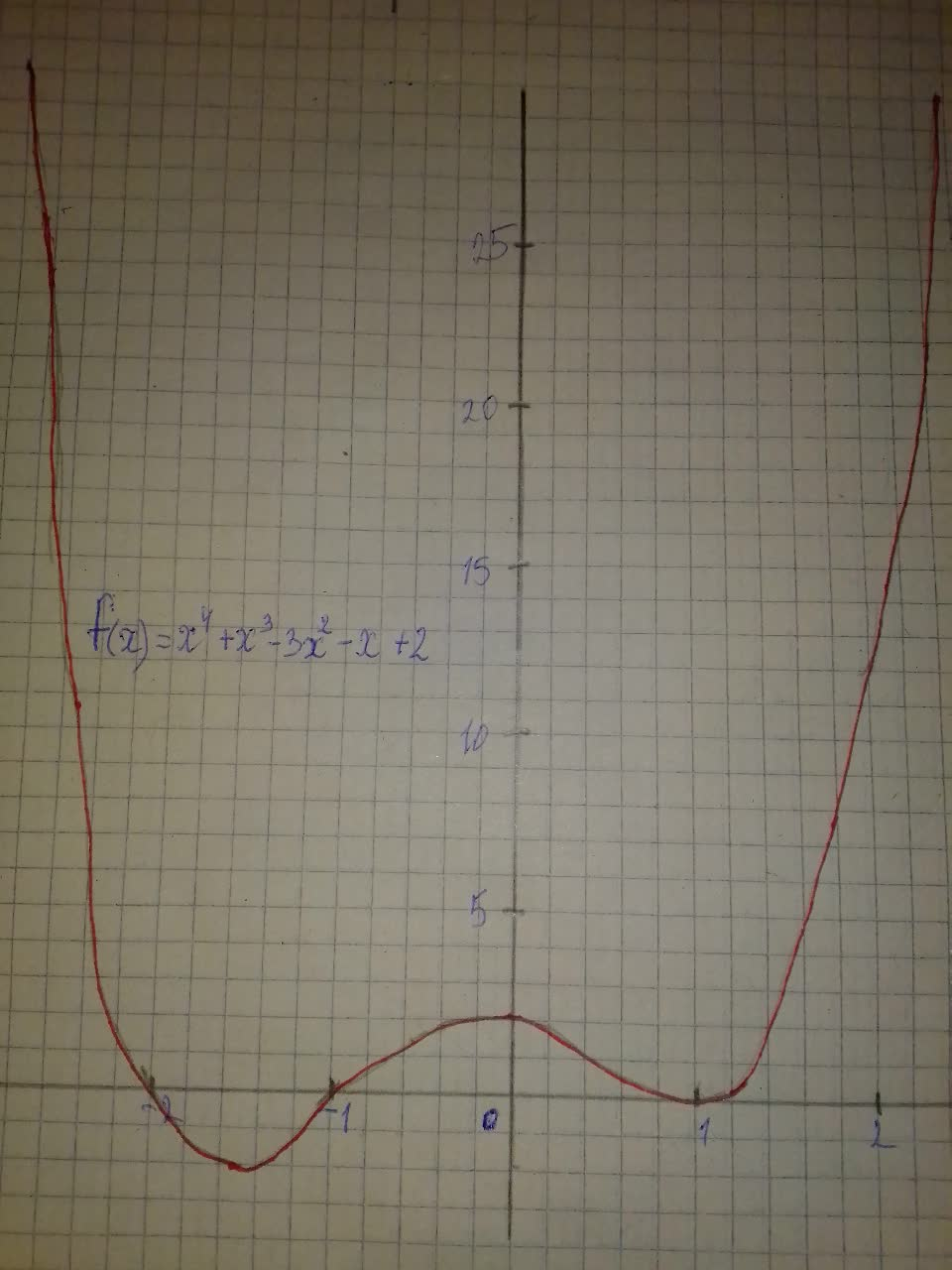Question# Graph each polynomial function f(x)=x^{4}+x^{3}-3x^{2}-x+2

Polynomial graphs
ANSWEREDGraph each polynomial function. $$\displaystyle{f{{\left({x}\right)}}}={x}^{{{4}}}+{x}^{{{3}}}-{3}{x}^{{{2}}}-{x}+{2}$$